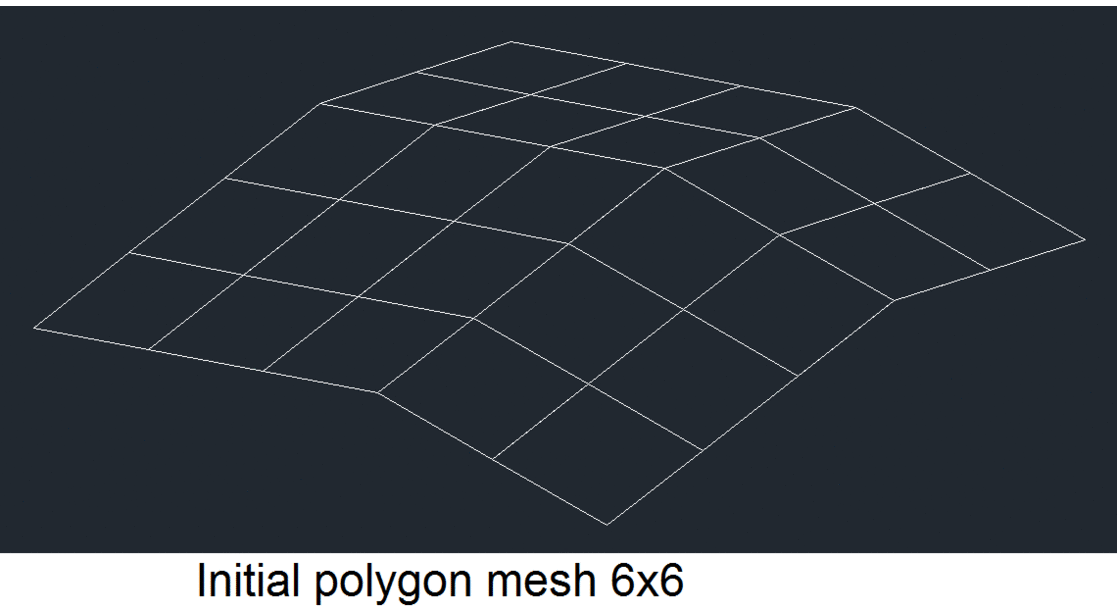# Working with Surface Fit Polygon Meshes

April 19, 2018

A polygon mesh can be of the surface fit type, which defines the polygon mesh using an approximation method to create an object that is more smooth. In Teigha, polygon mesh surface fit is provided by the following function:

``````void OdDbPolygonMesh::surfaceFit(OdDb::PolyMeshType surfType, OdInt16 surfu, OdInt16 surfv);
``````

The surface fits the polyline mesh using the surfType, surfU, and surfV argument values. Then the new vertices of the ‘k3dFitVertex’ type are calculated on that surface. This is the same as the common PEDIT command and its "SMOOTH SURFACE" option in other CAD systems, except Teigha’s method uses the control values that are passed in whereas the PEDIT command uses those in the database.

The parameter surfType can be one of the following:

• kCubicSurfaceMesh
• kBezierSurfaceMesh

This parameter selects the order (by both U and V) of a NURBS underlay surface which is used to compute “smoothed” mesh points. Note that for the kBezierSurfaceMesh option, the order of the NURBS is the mesh size by M and N respectively.

The parameters surfU and surfV define the number of rows and columns of the “smoothed” mesh.

#### Code example

``````OdDbPolygonMeshPtr pMesh = ...; // create some mesh
pMesh->surfaceFit(OdDb::kCubicSurfaceMesh, 12, 15);
for(OdDbObjectIteratorPtr pIter = pMesh->vertexIterator(); !pIter->done(); pIter->step())
{
OdDb::Vertex3dType type = pVert->vertexType();
if (type == OdDb::k3dFitVertex)
{
const OdGePoint3d pt = pVert->position();
... // use the fit vertex here
}
}
``````

Examples of initial mesh shapes and smooth results